# JP2011101266A - Semiconductor device and information processing system - Google Patents

## Info

Publication number
JP2011101266A
JP2011101266A JP2009255568A JP2009255568A JP2011101266A JP 2011101266 A JP2011101266 A JP 2011101266A JP 2009255568 A JP2009255568 A JP 2009255568A JP 2009255568 A JP2009255568 A JP 2009255568A JP 2011101266 A JP2011101266 A JP 2011101266A
Authority
JP
Japan
Prior art keywords
voltage
output
power supply
output driver
output signal
Prior art date
Legal status (The legal status is an assumption and is not a legal conclusion. Google has not performed a legal analysis and makes no representation as to the accuracy of the status listed.)
Withdrawn
Application number
JP2009255568A
Other languages
Japanese (ja)
Inventor
Yoji Nishio

Original Assignee
Elpida Memory Inc
エルピーダメモリ株式会社
Priority date (The priority date is an assumption and is not a legal conclusion. Google has not performed a legal analysis and makes no representation as to the accuracy of the date listed.)
Filing date
Publication date
Application filed by Elpida Memory Inc, エルピーダメモリ株式会社 filed Critical Elpida Memory Inc
Priority to JP2009255568A priority Critical patent/JP2011101266A/en
Publication of JP2011101266A publication Critical patent/JP2011101266A/en
Application status is Withdrawn legal-status Critical

• 239000004065 semiconductor Substances 0 abstract title 3
• 230000002829 reduced Effects 0 abstract 3
• 230000001965 increased Effects 0 abstract 1

## Images

•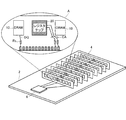•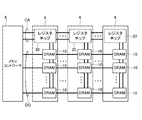•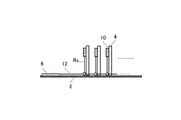•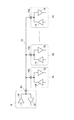•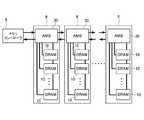•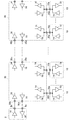•••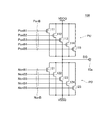••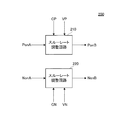•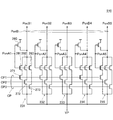••••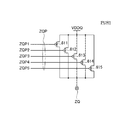•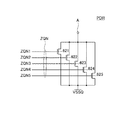•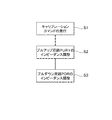•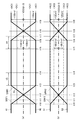•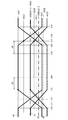•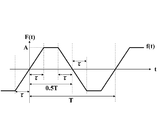•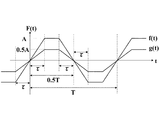•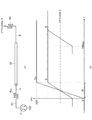•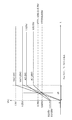••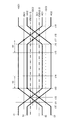•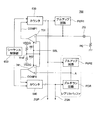•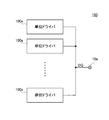•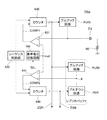•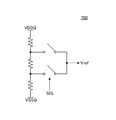•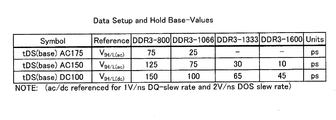•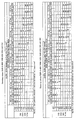•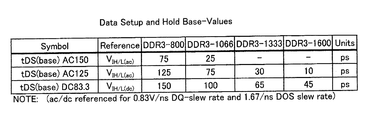•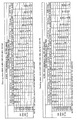•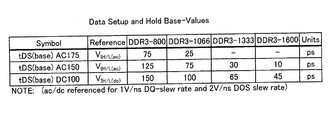•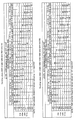••## Classifications

• HELECTRICITY
• H03BASIC ELECTRONIC CIRCUITRY
• H03KPULSE TECHNIQUE
• H03K19/00Logic circuits, i.e. having at least two inputs acting on one output; Inverting circuits
• H03K19/003Modifications for increasing the reliability for protection
• H03K19/00369Modifications for compensating variations of temperature, supply voltage or other physical parameters
• H03K19/00384Modifications for compensating variations of temperature, supply voltage or other physical parameters in field effect transistor circuits
• HELECTRICITY
• H03BASIC ELECTRONIC CIRCUITRY
• H03KPULSE TECHNIQUE
• H03K19/00Logic circuits, i.e. having at least two inputs acting on one output; Inverting circuits
• H03K19/003Modifications for increasing the reliability for protection
• H03K19/00346Modifications for eliminating interference or parasitic voltages or currents
• H03K19/00361Modifications for eliminating interference or parasitic voltages or currents in field effect transistor circuits

## Abstract

A semiconductor device capable of correctly transferring data even when a power supply voltage is lowered is provided.
An output driver and a characteristic switching circuit for switching characteristics of the output driver are provided. The characteristic switching circuit 18 uses the rise time and fall time of the output signal of the output driver 100 when the power supply voltage VDDQ supplied to the power supply line is the first voltage VDDQ1, and the power supply voltage VDDQ supplied to the power supply line. The rise time and fall time of the output signal of the output driver 100 in the case of the second voltage VDDQ2 are made to coincide with each other. As a result, even if the power supply voltage is lowered, the influence of harmonic components and crosstalk does not increase. Further, even if the power supply voltage is lowered, the reception condition on the receiver side does not change, so that it is possible to correctly transmit and receive signals regardless of the power supply voltage.
[Selection] Figure 7

## Description

The present invention relates to a semiconductor device and an information processing system, and more particularly to a semiconductor device having a variable power supply voltage and an information processing system including the same.

In a semiconductor device such as a DRAM (Dynamic Random Access Memory), the power supply voltage and the like are strictly determined by the standard for standardization. For example, in a DDR (Double Data Rate) type 3 SDRAM (Synchronous DRAM), the power supply voltage is regulated to 1.5 V (Non-Patent Document 1).

On the other hand, in recent years, since the demand for lower power consumption is very strong, many attempts have been made to lower the power supply voltage. However, if the power supply voltage is changed, the waveform of the output signal from the output driver also changes, so that transmission and reception of signals cannot be performed correctly. For this reason, various adjustments to the output driver and the input receiver are required. Generally, when the power supply voltage is lowered, the slew rate of the output driver is designed to maintain the slew rate before the power supply voltage is lowered.

1G bits DDR3 SDRAM (Document No.E1494E50 (Ver. 5.0)) URL http://www.elpida.com/pdfs/E1494E50.pdf

However, when the power supply voltage is lowered, the amplitude of the output signal generally becomes smaller. Therefore, when the slew rate is designed to maintain the slew rate before the power supply voltage is lowered, the harmonic component of the signal waveform increases and The effect of talk is also increased. As a result, since the signal propagation waveform is disturbed, it is difficult to obtain good signal quality at a high data transfer rate.

The present inventor noted that the frequency component of the signal waveform does not change if the output signal rise time and fall time are maintained instead of maintaining the slew rate of the output driver when the power supply voltage is lowered. The semiconductor device which realizes this has been studied earnestly. The present invention has been made based on such technical knowledge.

A semiconductor device according to one aspect of the present invention is connected to a power supply line, connected to an external terminal, an output driver that outputs an output signal to the external terminal, a characteristic switching circuit that switches a characteristic of the output signal of the output driver, The characteristic switching circuit includes a calibration circuit that controls output impedance when the output driver is conductive, a slew rate control circuit that adjusts a slew rate indicating a potential transition amount per unit time of the output signal, and The calibration circuit includes the case where the power supply voltage supplied to the power supply line is a first voltage and the case where the power supply voltage is a second voltage smaller than the first voltage. The slew rate control is performed by matching output impedances when the output drivers corresponding to the first and second voltages are turned on. When the path is the first voltage, the slew rate of the output signal is relatively increased, and the amplitude value of the output signal is set to the first amplitude value corresponding to the first voltage. Thus, the rise time and fall time of the output signal are first and second times, respectively, and when the voltage is the second voltage, the slew rate of the output signal is relatively reduced, and By setting the amplitude value of the output signal to a second amplitude value corresponding to the second voltage smaller than the first amplitude value, the rise time and fall time of the output signal are set to the first and It is set as the 2nd time.

A semiconductor device according to another aspect of the present invention is connected to a power supply line, connected to an external terminal, and outputs an output signal to the external terminal; and a characteristic switching circuit that switches the characteristics of the output signal of the output driver; The characteristic switching circuit includes: a calibration circuit that controls an output impedance when the output driver is conductive; and a slew rate control circuit that adjusts a slew rate indicating a potential transition amount per unit time of the output signal; The calibration circuit includes a case where a power supply voltage supplied to the power supply line is a first voltage and a case where the power supply voltage is a second voltage smaller than the first voltage. The output impedance of the output driver corresponding to the second voltage when the output driver is conductive is that of the output driver corresponding to the first voltage. The slew rate control circuit matches the slew rates of the output signals corresponding to the first and second voltages, respectively, when the first and second voltages are used. And by causing the first and second amplitude values of the output signal corresponding to the first and second voltages to coincide with each other, the rising edge of the output signal of the output driver in the case of the first voltage The time and the fall time are respectively the first and second times, and the rise time and the fall time of the output signal of the output driver in the case of the second voltage are the first and second times, respectively. It is characterized by that.

An information processing system according to an aspect of the present invention includes a first semiconductor device having a first output driver connected to a first power supply line, and a first input receiver connected to a second power supply line. An information processing system comprising: a second semiconductor device including: a data wiring that transmits an output signal output from the first output driver to the first input receiver; wherein the first semiconductor device includes: A first slew rate control circuit that adjusts a slew rate indicating a potential transition amount per unit time of an output signal output from the first output driver, wherein the first slew rate control circuit includes the first slew rate control circuit. When the power supply voltage supplied to the power supply line is the first voltage, the rise time and the fall time of the output signal of the first output driver are the first time and the second time, respectively. And the rise time and fall time of the output signal of the first output driver when the power supply voltage supplied to the first power supply line is a second voltage lower than the first voltage, respectively. The slew rate of the output signal of the first output driver corresponding to the second voltage is set to be the first and second times than the slew rate of the output signal of the first output driver corresponding to the first voltage. The impedance of the first output driver corresponding to each of the first and second voltages is made smaller regardless of the first and second voltages, and further corresponds to the first and second voltages, respectively. The ratio of the amplitude value of the output signal of the first output driver is made to correspond to the ratio of the first and second voltages, or the first output driver corresponding to the first and second voltages, respectively. The slew rates of the output signals of the first and second output drivers are matched, and the impedance of the first output driver corresponding to the second voltage is made lower than the impedance of the first output driver corresponding to the first voltage. The amplitude value of the output signal of the first output driver corresponding to each of the first and second voltages is matched regardless of the first and second voltages.

An information processing system according to another aspect of the present invention is an information processing system including an output driver, an input receiver that receives an output signal output from the output driver, and a control circuit that controls the output driver. A first operating condition in which the power supply voltage supplied to the output driver is a first voltage that is relatively high; and a second operating condition in which the power supply voltage is a second voltage that is relatively lower than the first voltage. And the control circuit determines the slew rate in the second operating condition indicating the potential transition amount per unit time of the output signal output from the output driver, as the first operating condition. The rise time of the output signal under the first operating condition is made smaller than the slew rate indicating the potential transition amount per unit time output by the output driver And the fall time and the rise time and fall time of the output signal in the second operating condition are made to coincide with each other, and the output impedance of the output driver and the second operating condition in the first operating condition And the output impedance of the output driver in the first operation condition and the ratio of the amplitude value of the output signal in the second operation condition to the first operation condition. The power supply voltage in a condition is proportional to the ratio of the power supply voltage in the second operating condition.

An information processing system according to still another aspect of the present invention is an information processing system including an output driver, an input receiver that receives an output signal output from the output driver, and a control circuit that controls the output driver. A first operating condition in which the power supply voltage supplied to the output driver is a relatively high first voltage, and the power supply voltage is a second voltage that is relatively lower than the first voltage. The control circuit has a second operation condition, and the control circuit matches a slew rate in each of the first and second operation conditions indicating a potential transition amount per unit time of an output signal output from the output driver. The rise time and fall time of the output signal under the first operating condition, and the rise time and fall time of the output signal under the second operating condition. And the output driver's output impedance in the second operating condition is lower than the output impedance of the output driver in the first operating condition, and the output driver's output impedance in the first operating condition is further reduced. The amplitude value of the output signal and the amplitude value of the output signal under the second operating condition are matched regardless of the first and second voltages.

According to the present invention, since the rise time and fall time of the output signal are maintained by the characteristic switching circuit, the influence of harmonic components, crosstalk, and reflection does not increase even if the power supply voltage is lowered. . In addition, even if the power supply voltage is lowered, the reception condition on the receiver side can be made compatible with this, so that it is possible to correctly transmit and receive signals regardless of the power supply voltage. In addition, power consumption can be reduced.

1 is a schematic perspective view schematically showing the structure of a memory system to which the present invention is applied. FIG. 2 is a block diagram of the memory system shown in FIG. 1. FIG. 2 is a schematic side view of the memory system shown in FIG. 1. FIG. 2 is a circuit diagram showing extracted elements related to transmission / reception of data DQ in the memory system shown in FIG. 1. It is a block diagram of a memory system using FBDIMM. FIG. 6 is a circuit diagram showing extracted elements related to transmission / reception of data DQ in the memory system shown in FIG. 5. 1 is a block diagram showing a configuration of an output circuit 14 according to a first embodiment of the present invention. (A) is a block diagram showing the register 410 to which the switching signal SEL is set, and (b) is a block diagram of the voltage detection circuit 420. 2 is a circuit diagram of the output driver 100. FIG. It is a circuit diagram of the output driver 100 by a modification. 2 is a block diagram of a slew rate control circuit 200. FIG. 3 is a circuit diagram of a slew rate adjustment circuit 210. FIG. 3 is a circuit diagram of a slew rate adjustment circuit 220. FIG. 2 is a circuit diagram of an output control circuit 500. FIG. 2 is a circuit diagram of a calibration circuit 600. FIG. It is a circuit diagram of pull-up circuit PUR1. It is a circuit diagram of a pull-down circuit PDR. 5 is a flowchart showing the operation of a calibration circuit 600. It is a wave form diagram of data DQ, (a) shows the case where power supply voltage VDDQ is the 1st voltage VDDQ1 (1.5V), and (b) shows power supply voltage VDDQ being the 2nd voltage VDDQ2 (1.25V). The case is shown. FIG. 20 is a diagram in which FIG. 19A and FIG. It is a waveform model of a periodic trapezoidal wave. It is a waveform model of a periodic trapezoidal wave. (A) is a model figure of a transmission system, (b) is a schematic diagram which shows the signal waveform of each point. It is a schematic diagram which shows the signal waveform of each point. It is a block diagram which shows the structure of the output circuit 14a by the 2nd Embodiment of this invention. FIG. 10 is a waveform diagram of data DQ output from an output driver 100 in the second embodiment. 2 is a block diagram of a calibration circuit 700. FIG. It is a block diagram of the output driver 100 which consists of several unit drivers 100a. It is a block diagram of the calibration circuit 700a. 6 is a circuit diagram of a reference potential switching circuit 760. FIG. 6 is a table showing receiver characteristics (setup time and hold time) when the power supply voltage VDDQ is the first voltage VDDQ1 in the first embodiment. 5 is a table showing receiver characteristics (De-rating Value) when the power supply voltage VDDQ is the first voltage VDDQ1 in the first embodiment. 6 is a table showing receiver characteristics (setup time and hold time) when the power supply voltage VDDQ is the second voltage VDDQ2 in the first embodiment. 6 is a table showing receiver characteristics (De-rating Value) when the power supply voltage VDDQ is the second voltage VDDQ2 in the first embodiment. 10 is a table showing receiver characteristics (setup time and hold time) when the power supply voltage VDDQ is the first voltage VDDQ1 in the second embodiment. 10 is a table showing receiver characteristics (De-rating Value) when the power supply voltage VDDQ is the first voltage VDDQ1 in the second embodiment. 10 is a table showing receiver characteristics (setup time and hold time) when the power supply voltage VDDQ is the second voltage VDDQ2 in the second embodiment. 10 is a table showing receiver characteristics (De-rating Value) when the power supply voltage VDDQ is the second voltage VDDQ2 in the second embodiment.

A typical example of a technical idea (concept) for solving the problems of the present invention is shown below. However, it goes without saying that the claimed contents of the present application are not limited to this technical idea, but are the contents described in the claims of the present application. That is, the present invention pays attention to the fact that the frequency component of the signal waveform does not change if the rise time and fall time of the output signal do not change even if the power supply voltage is changed, and by controlling the slew rate or amplitude. The technical idea is to make the rise time and fall time of the output signal constant regardless of the power supply voltage. Here, the “slew rate” refers to the amount of signal transition (transition) per unit time.

Hereinafter, preferred embodiments of the present invention will be described in detail with reference to the accompanying drawings.

FIG. 1 is a schematic perspective view schematically showing the structure of a memory system to which the present invention is applied.

The memory system shown in FIG. 1 is a memory system using a so-called RDIMM (Registered Dual Inline Memory Module), and is configured to be able to switch the level of a power supply voltage VDDQ used for driving a bus line in two stages. The first voltage VDDQ1 is a relatively high voltage, for example, 1.5V. The second voltage VDDQ2 is a relatively low voltage, for example, 1.25V. As described above, the memory system according to the present embodiment can be used by setting the power supply voltage VDDQ used for driving the bus line to VDDQ1 (1.5V), or set to VDDQ2 (1.25V). Is also possible. The operation associated with the switching of the power supply voltage VDDQ will be described later. Here, the power supply voltage VDDQ is a power supply voltage of a different system different from the power supply voltage VDD serving as an operation power supply for the internal circuit. However, the levels of the power supply voltages VDD and VDDQ may be the same. To be precise, the “power supply voltage VDDQ” indicates a potential difference between the power supply potential VDDQ on the higher side and the power supply potential VSSQ on the lower side.

As shown in FIG. 1, the memory system according to the present embodiment has a configuration in which a plurality of RDIMMs 4 are mounted on a motherboard 2. These RDIMMs 4 are connected to a memory controller 6 mounted on the motherboard 2. As shown in the enlarged portion A of FIG. 1, the RDIMM 4 includes a register chip 20 in addition to the plurality of DRAMs 10. The command address signal CA other than the data DQ and the data strobe signals DQS and / DQS is not directly input to the DRAM 10 but is buffered by the register chip 20 and then supplied to each DRAM 10.

On the other hand, the data DQ and the data strobe signals DQS and / DQS are directly transmitted and received between the memory controller 6 and each DRAM 10 without going through the register chip 20. Reference sign Rs shown in FIG. 1 is a stub resistor. In the following description, the data DQ and the data strobe signals DQS and / DQS may be simply referred to as “data DQ” unless there is a particular need for distinction.

As shown in FIGS. 2 and 3, each RDIMM 4 is commonly connected to the memory controller 6. FIG. 3 shows a state in which the data wiring 12 provided on the mother board 2 is commonly connected to the corresponding DRAM 10 of each RDIMM 4 via the stub resistor Rs. As described above, in the RDIMM system, the DRAM 10 and the register chip 20 are commonly connected between a plurality of RDIMMs.

FIG. 4 is a circuit diagram showing extracted elements related to transmission / reception of data DQ. As shown in FIG. 4, the data input / output terminals 6 a and 10 a provided in the memory controller 6 and the DRAM 10 are commonly connected via the data wiring 12. The memory controller 6 and the DRAM 10 include an output circuit 14 and an input circuit 16 connected to data input / output terminals 6a and 10a, respectively. As a result, during the read operation, the data DQ is output from the output circuit 14 of any DRAM 10 and is received by the input circuit 16 in the memory controller 6. On the other hand, during the write operation, the data DQ is output from the output circuit 14 in the memory controller 6 and is received by the input circuit 16 in any DRAM 10. The feature of the present invention resides in these output circuits 14, and details thereof will be described later.

Even when the present invention is applied to a memory system, it goes without saying that the memory module to be used is not limited to RDIMM, FBDIMM (Fully Buffered DIMM), UDIMM (Unbuffered DIMM), LRDIMM (Load Reduced DIMM), etc. It is also possible to use.

As shown in FIG. 5, the FBDIMM 8 is a type of DIMM that receives all signals such as an address signal, a data signal, and a control signal by a chip 30 called an AMB (Advanced Memory Buffer) once mounted on a module substrate. The AMB 30 may be mounted on the motherboard 2. The AMB 30 is an LSI chip having a parallel-serial conversion function. That is, the AMB 30 and the memory controller 6 are connected by a point-to-point serial interface similar to PCI Express. On the other hand, the AMB 30 and the DRAM 10 are connected by a conventional parallel interface. The AMB 30 converts the serially issued memory control commands into parallel and transfers them to the DRAM 10 collectively. Data DQ is transferred bidirectionally.

FIG. 6 is a circuit diagram showing extracted elements related to transmission / reception of data DQ in the FBDIMM system. As shown in FIG. 6, the memory controller 6 and the plurality of AMBs 30 are connected in cascade, and write data is transferred via the data output terminal 6b, the data input terminal 30b, and the data output terminal 30c at the time of writing. In reading, read data is transferred via the data output terminal 30d, the data input terminal 30e, and the data input terminal 6c. On the other hand, the AMB 30 and the DRAM 10 are individually connected via data input / output terminals 10a and 30a. The output circuit 14 and the input circuit 16 are used for data transfer. As described above, the output circuit 14 is a feature of the present invention.

Although not shown, the UDIMM is a DIMM in a form in which an address signal, a control signal, and a data signal from the memory controller 6 are distributed and connected directly to the DRAM 10 on the module substrate. Unlike the RDIMM and FBDIMM, the register chip 20 and the AMB 30 are not interposed between the memory controller 6 and the DRAM 10, which is advantageous in terms of latency. However, since the electrical load seen from both the memory controller 6 and the DRAM 10 is large, it is not suitable for connecting many DIMMs. Although not shown, in the LRDIMM, the register chip 20 of the RDIMM is replaced with a memory buffer. In the RDIMM, the data DQ is directly exchanged between the memory controller 6 and the DRAM 10. In the LRDIMM, the data DQ is exchanged between the memory controller 6 and the DRAM 10 via the memory buffer. The exchange of data DQ signals between the memory buffer and the DRAM 10 in the LRDIMM is a point-to-point topology and is the same as in the FBDIMM.

Hereinafter, the configuration of the output circuit 14 shown in FIGS. 4 and 6 will be described in more detail.

FIG. 7 is a block diagram showing a configuration of the output circuit 14 according to the first embodiment of the present invention.

As shown in FIG. 7, the output circuit 14 according to the present embodiment includes an output driver 100 that drives the data input / output terminal 10a, and a characteristic switching circuit 18 that switches the characteristics of the output driver 100 based on the power supply voltage VDDQ. Yes. The characteristic switching circuit 18 includes a slew rate control circuit 200 that adjusts the slew rate of the output driver 100. The output driver 100 outputs high-level or low-level data DQ from the data input / output terminal 10a based on the ON signals NonB and PonB. Although not particularly limited, in the present embodiment, the ON signals NonB and PonB are both 5-bit signals. This is because, as will be described later, both the pull-up circuit PU and the pull-down circuit PD included in the output driver 100 are configured by five output transistors. If the output circuit 14 has an ODT (On Die Termination) function, the output driver 100 can also function as a termination resistor. A specific circuit configuration of the output driver 100 will be described later.

The slew rate control circuit 200 is a circuit that receives the on signals PonA and NonA, the slew rate setting signals CP and CN, and the source potentials VP and VN and generates the on signals PonB and NonB. The on signal PonB is logically an inverted signal of the on signal PonA, but its waveform and level are adjusted by the slew rate setting signal CP and the source potential VP. Similarly, the ON signal NonB is logically an inverted signal of the ON signal NonA, but its waveform and level are adjusted by the slew rate setting signal CN and the source potential VN. A specific circuit configuration of the slew rate control circuit 200 will be described later.

The slew rate setting signals CP and CN are signals output from the slew rate setting circuit 300, and are not particularly limited, but are each a 3-bit signal in the present embodiment. The values of the slew rate setting signals CP and CN are fixed by performing nonvolatile writing (for example, destruction of the fuse element or antifuse element) to the slew rate setting circuit 300 in the manufacturing stage. Writing to the slew rate setting circuit 300 in the manufacturing stage is performed based on the result of an operation test performed in a wafer state, the use of the semiconductor chip, and the like. Therefore, the slew rate set by the slew rate setting signals CP and CN is an optimum value at the time of shipment. However, in the present invention, the slew rate setting signals CP and CN are not necessarily fixed values, and may be variable.

The source potentials VP and VN are potentials supplied from the source potential adjustment circuit 400. The switching signal SEL is input to the source potential adjusting circuit 400, and the levels of the generated source potentials VP and VN are selected by the switching signal SEL. The switching signal SEL is a signal indicating whether the power supply voltage VDDQ is a relatively high voltage VDDQ1 (1.5 V) or a relatively low voltage VDDQ2 (1.25 V). As shown in FIG. 8A, the switching signal SEL is acquired by referring to the setting value of the register 410 indicating whether the power supply voltage VDDQ is the first level VDDQ1 or the second level VDDQ2. Alternatively, as shown in FIG. 8B, the voltage detection circuit 420 that detects the level of the power supply voltage VDDQ may be used, and the detection result of the voltage detection circuit 420 may be referred to. In the present embodiment, since the level of the power supply voltage VDDQ is set to VDDQ1 (1.5V) or VDDQ2 (1.25V), a 1-bit digital signal is sufficient for the switching signal SEL. For example, when the level of the power supply voltage VDDQ is VDDQ1 (1.5 V), the switching signal SEL is at a high level, and when the level of the power supply voltage VDDQ is VDDQ2 (1.25 V), the switching signal SEL is Become low level. However, the present invention is not limited to this. For example, when the voltage detection circuit 420 shown in FIG. 8B is used, the level detection of the power supply voltage VDDQ may be stepless and the switching signal SEL may be an analog value.

The on signals PonA and NonA are signals supplied from the output control circuit 500, and in the present embodiment, each is a 5-bit signal, similar to the on signals PonB and NonB. The output control circuit 500 receives internal data P and N and impedance adjustment signals ZQP and ZQN supplied from an internal circuit (not shown), and generates on signals PonA and NonA. A specific circuit configuration of the output control circuit 500 will be described later.

The impedance adjustment signals ZQP and ZQN are signals supplied from the calibration circuit 600. Although details will be described later, the calibration circuit 600 includes a replica buffer having substantially the same circuit configuration as that of the output driver 100. By performing a calibration operation using the replica buffer, an impedance adjustment signal is obtained. ZQP and ZQN are generated. Among these, the impedance adjustment signal ZQP is a signal for adjusting the pull-up circuit included in the output driver 100, and the impedance adjustment signal ZQN is a signal for adjusting the pull-down circuit. In the present embodiment, the impedance adjustment signals ZQP and ZQN are both 5-bit signals.

Next, each circuit constituting the output circuit 14 shown in FIG. 7 will be described in detail.

FIG. 9 is a circuit diagram of the output driver 100.

As shown in FIG. 9, the output driver 100 includes a plurality of (five in this embodiment) P-channels connected in parallel between the power supply line to which the higher power supply potential VDDQ is supplied and the data input / output terminal 10a. A plurality (five in this embodiment) of N-channel MOS transistors connected in parallel between the output transistors 111 to 115 made of MOS transistors and the data output terminal 10a and the power supply line to which the lower power supply potential VSSQ is supplied. Output transistors 121 to 125. In the output driver 100, a parallel circuit composed of the output transistors 111 to 115 constitutes a pull-up circuit PU, and a parallel circuit composed of the output transistors 121 to 125 constitutes a pull-down circuit PD.

Five operation signals PonB1 to PonB5 constituting the ON signal PonB are supplied to the gates (control electrodes) of the output transistors 111 to 115, respectively. Accordingly, the output transistors 111 to 115 constituting the pull-up circuit PU are individually controlled to be on (electrically conductive) / off (electrically nonconductive) based on the operation signals PonB1 to PonB5. Similarly, five operation signals NonB1 to NonB5 constituting the ON signal NonB are supplied to the gates (control electrodes) of the output transistors 121 to 125, respectively. Therefore, the output transistors 121 to 125 constituting the pull-down circuit PD are individually controlled to be turned on / off based on the operation signals NonB1 to NonB5. In the following description, when a transistor is electrically conductive or conductive is simply referred to as “on”, and when a transistor is electrically non-conductive or non-conductive is simply referred to as “off”. is there.

The pull-up circuit PU and the pull-down circuit PD constituting the output driver 100 are designed to have a predetermined impedance when conducting. However, the on-resistance of the transistor (impedance value when the transistor is electrically conductive) varies depending on the manufacturing conditions and varies depending on the environmental temperature and the power supply voltage during operation. Therefore, a desired impedance is not always obtained. Absent. Therefore, in order to set the actual impedance to a desired value, it is necessary to adjust the number of transistors to be turned on. For this purpose, a parallel circuit including a plurality of output transistors is used.

In order to finely adjust the impedance of the output driver 100 over a wide range, the W / L ratio (gate width / gate length ratio) of the plurality of output transistors constituting the pull-up circuit PU and the pull-down circuit PD may be different from each other. It is particularly preferable to weight the power of 2. That is, when the W / L ratio of the output transistor 111 is “1WLp”, the W / L ratios of the output transistors 112 to 115 can be set to “2WLp”, “4WLp”, “8WLp”, and “16WLp”, respectively. Particularly preferred. Similarly, when the W / L ratio of the output transistor 121 is “1WLn”, the W / L ratios of the output transistors 122 to 125 are set to “2WLn”, “4WLn”, “8WLn”, and “16WLn”, respectively. Is particularly preferred.

With such a configuration, by appropriately selecting output transistors that are turned on by the operation signals PonB1 to PonB5, NonB1 to NonB5, the impedances of the pull-up circuit PU and the pull-down circuit PD can be set regardless of variations due to manufacturing conditions or temperature changes. A desired value can be obtained.

However, the configuration of the output driver 100 is not limited to the circuit shown in FIG. 9. For example, as shown in FIG. 10, a resistor R is inserted between the data input / output terminal 10a and the pull-up circuit PU and pull-down circuit PD. It doesn't matter. As such a resistor R, for example, a tungsten (W) resistor can be used.

FIG. 11 is a block diagram of the slew rate control circuit 200.

As shown in FIG. 11, the slew rate control circuit 200 includes a slew rate adjustment circuit 210 that generates an on signal PonB and a slew rate adjustment circuit 220 that generates an on signal NonB. The slew rate adjusting circuit 210 is a circuit that converts the on signal PonA into the on signal PonB based on the slew rate setting signal CP and the source potential VP. Similarly, the slew rate adjusting circuit 220 is a circuit that converts the on signal NonA into the on signal NonB based on the slew rate setting signal CN and the source potential VN.

As described above, the ON signal PonB is logically an inverted signal of the ON signal PonA, but its waveform and level are adjusted by the slew rate setting signal CP and the source potential VP. Similarly, the ON signal NonB is logically an inverted signal of the ON signal NonA, but its waveform and level are adjusted by the slew rate setting signal CN and the source potential VN.

FIG. 12 is a circuit diagram of the slew rate adjustment circuit 210.

As shown in FIG. 12, the slew rate adjusting circuit 210 includes drive circuits 231 to 235 that generate on signals PonB1 to PonB5 based on the on signals PonA1 to PonA5, respectively. The drive circuit 231 includes N-channel MOS transistors (selection transistors) 261 to 263 to which an on signal PonA1 is supplied and N-channel MOS transistors (adjustment transistors) 271 to 273 to which slew rate setting signals CP1 to CP3 are supplied. Each of them has a configuration in which three series circuits are connected in parallel. The slew rate setting signals CP1 to CP3 are signals constituting the slew rate setting signal CP.

When the bits CP1 to CP3 constituting the slew rate setting signal CP are weighted, at least the adjustment transistors 271 to 273 have different W / L ratios (gate width / gate length ratio) according to the weights. Preferably. Specifically, when the weights of the slew rate settings CP1 to CP3 are “1”, “2”, and “4”, respectively, when the W / L ratio of the adjustment transistor 271 is “1 WLps”, the adjustment transistor The W / L ratios of 272 and 273 may be set to “2 WLps” and “4 WLps”, respectively.

The source potential VP supplied from the source potential adjustment circuit 400 is supplied to the source of the drive circuit 231. The source potential adjustment circuit 400 sets the source potential VP to VSS when the switching signal SEL is at a high level (VDDQ = VDDQ1), and when the switching signal SEL is at a low level (VDDQ = VDDQ2), The source potential VP is set to a negative potential VSS2 less than VSS.

The other drive circuits 232 to 235 also have the same circuit configuration as the drive circuit 231 except that the on signals PonA2 to PonA5 are supplied.

With this circuit configuration, for the drive circuits 231 to 235 in which the corresponding on signals PonA1 to PonA5 are at the active level (high level), the on signals PonB1 to PonB5 that are the outputs are activated to the low level (VSS or VSS2). . The on signals PonB1 to PonB5 are reset to a high level by the P-channel MOS transistor 260 when the on signals PonA1 to PonA5 return to the inactive level (low level).

The waveforms and levels of the on signals PonB1 to PonB5 based on the activation of the on signals PonA1 to PonA5 are adjusted by the slew rate setting signal CP and the source potential VP. Specifically, when the slew rate setting signal CP designates a higher slew rate, the fall of the on signals PonB1 to PonB5 becomes steeper, and conversely, the slew rate setting signal CP has a lower slew rate. Is specified, the fall of the ON signals PonB1 to PonB5 becomes more gradual. When the power supply voltage VDDQ is set to VDD2 (1.25 V), the source potential VP is set to VSS2 (<VSS), so that the slew rate is compared with the case where the source potential VP is VSS. Is increased.

The ON signals PonB1 to PonB5 generated in this way are supplied to the pull-up circuit PU of the output driver 100 shown in FIG. Since the pull-up circuit PU is composed of P-channel MOS transistors 111 to 115, the on-resistance (output impedance) varies depending on the gate-source voltage (Vgs) and the source-drain voltage (Vds). To do. Therefore, the on-resistance when the power supply voltage VDDQ is relatively low VDD2 (1.25 V) is higher than the on-resistance when the power supply voltage VDDQ is relatively high VDD1 (1.5 V). . In the present specification, the output impedance indicates the impedance when the output driver 100 (pull-up circuit PU or pull-down circuit PD) is electrically conductive. Further, in the present specification, the impedance value is simply referred to as “impedance”.

However, in this embodiment, when the power supply voltage VDDQ is set to VDD2 (1.25 V), the source potential VP is switched to VSS2 lower than VSS, so that the on-state when the power supply voltage VDDQ is VDD1 is switched on. The resistance and the on-resistance when the power supply voltage VDDQ is VDD2 are equal. More precisely, the level of VSS2 is determined so that the on-resistance when the power supply voltage VDDQ is VDD1 and the on-resistance when the power supply voltage VDDQ is VDD2 are equal.

FIG. 13 is a circuit diagram of the slew rate adjustment circuit 220.

As shown in FIG. 13, the slew rate adjustment circuit 220 includes drive circuits 241 to 245 that generate the on signals NonB1 to NonB5 based on the on signals NonA1 to NonA5, respectively. The drive circuit 241 includes N-channel MOS transistors (select transistors) 281 to 283 to which an on signal NonA1 is supplied and N-channel MOS transistors (adjustment transistors) 291 to 293 to which slew rate setting signals CN1 to CN3 are supplied. Each of them has a configuration in which three series circuits are connected in parallel. The slew rate setting signals CN1 to CN3 are signals constituting the slew rate setting signal CN.

When the bits CN1 to CN3 constituting the slew rate setting signal CN are weighted, at least the adjustment transistors 291 to 293 have different W / L ratios (gate width / gate length ratio) according to the weights. Preferably. Specifically, when the weights of the slew rate settings CN1 to CN3 are “1”, “2”, and “4”, respectively, when the W / L ratio of the adjustment transistor 291 is “1WLns”, the adjustment transistor The W / L ratios of 292 and 293 may be set to “2WLns” and “4WLns”, respectively.

The source potential VN supplied from the source potential adjustment circuit 400 is supplied to the source of the drive circuit 241. When the switching signal SEL is at a high level (VDDQ = VDDQ1), the source potential adjustment circuit 400 sets the source potential VN to VDD, and when the switching signal SEL is at a low level (VDDQ = VDDQ2), The source potential VN is set to a boosted potential VDD2 that exceeds VDD.

The other drive circuits 242 to 245 have the same circuit configuration as the drive circuit 241 except that the ON signals NonA2 to NonA5 are supplied.

With this circuit configuration, for the drive circuits 241 to 245 whose corresponding ON signals NonA1 to NonA5 are at the active level (low level), the ON signals NonB1 to NonB5 that are the outputs are activated to the high level (VDD or VDD2). . The on signals NonB1 to NonB5 are reset to a low level by the N-channel MOS transistor 280 when the on signals NonA1 to NonA5 return to the inactive level (high level).

The waveforms and levels of the on signals NonB1 to NonB5 based on the activation of the on signals NonA1 to NonA5 are adjusted by the slew rate setting signal CN and the source potential VN. Specifically, when the slew rate setting signal CN designates a higher slew rate, the rise of the on signals NonB1 to NonB5 becomes steeper, and conversely, the slew rate setting signal CN has a lower slew rate. When designated, the rise of the ON signals NonB1 to NonB5 becomes more gradual. Further, when the power supply voltage VDDQ is set to VDD2 (1.25 V), the source potential VN is set to VDD2 (> VDD), so that the slew rate is compared with the case where the source potential VN is VDD. Is increased.

The ON signals NonB1 to NonB5 generated in this way are supplied to the pull-down circuit PD of the output driver 100 shown in FIG. Since the pull-down circuit PD includes N-channel MOS transistors 121 to 125, the on-resistance (output impedance) varies depending on the gate-source voltage (Vgs) and the source-drain voltage (Vds). . Therefore, the on-resistance when the power supply voltage VDDQ is relatively low VDD2 (1.25 V) is higher than the on-resistance when the power supply voltage VDDQ is relatively high VDD1 (1.5 V). .

However, in this embodiment, when the power supply voltage VDDQ is set to VDD2 (1.25 V), the source potential VN is switched to VDD2 higher than VDD, so that the on-state when the power supply voltage VDDQ is VDD1 is switched on. The resistance and the on-resistance when the power supply voltage VDDQ is VDD2 are equal. More precisely, the level of VDD2 is determined so that the on-resistance when the power supply voltage VDDQ is VDD1 and the on-resistance when the power supply voltage VDDQ is VDD2 are equal.

FIG. 14 is a circuit diagram of the output control circuit 500.

As shown in FIG. 14, the output control circuit 500 includes five NOR circuits 511 to 515 and five NAND circuits 521 to 525. The NOR circuits 511 to 515 are supplied with internal data P in common and impedance adjustment signals ZQP1 to ZQP5 constituting the impedance adjustment signal ZQP, respectively. On the other hand, internal data N is commonly supplied to the NAND circuits 521 to 525, and impedance adjustment signals ZQN1 to ZQN5 constituting the impedance adjustment signal ZQN are supplied to the NAND circuits 521 to 525, respectively.

The internal data P and N are signals indicating the logical value of the data to be output. If the data to be output from the data input / output terminal 10a is at the high level, both are set to the low level and output from the data input / output terminal 10a. If the data to be processed is at a low level, all are set to a high level. Therefore, the internal data P and N can be a single signal. However, when the output driver 100 performs the ODT operation, it is necessary to set the internal data P to the low level and the internal data N to the high level. In the present embodiment, a separate signal is used assuming such a case. As described above, the internal data P and N are signals generated by an internal circuit (not shown).

With such a configuration, when the internal data P and N are at the low level, at least one of the operation signals PonA1 to PonA5 that are the outputs of the NOR circuits 511 to 515 is activated to the high level, while the NAND circuits 521 to The operation signals NonA1 to NonA5, which are the outputs of 525, are all deactivated to a high level. In this case, which of the operation signals PonA1 to PonA5 is activated is determined by the impedance adjustment signal ZQP. The operation signals PonA1 to PonA5 constitute the operation signal PonA shown in FIG.

Similarly, when the internal data P and N are at the high level, at least one of the operation signals NonA1 to NonA5 which are the outputs of the NAND circuits 521 to 525 is activated to the low level, while the outputs of the NOR circuits 511 to 515 are activated. The operation signals PonA1 to PonA5 are all deactivated to a low level. In this case, which of the operation signals NonA1 to NonA5 is activated is determined by the impedance adjustment signal ZQN. The operation signals NonA1 to NonA5 constitute the operation signal NonA shown in FIG.

FIG. 15 is a circuit diagram of the calibration circuit 600.

As shown in FIG. 15, the calibration circuit 600 includes a pull-up circuit PUR1, PUR2, a pull-down circuit PDR, a counter 630 that controls the operation of the pull-up circuits PUR1, PUR2, and a counter that controls the operation of the pull-down circuit PDR. 640, comparators 631 and 641 for controlling the counters 630 and 640, and a sequence control unit 650 for controlling the operations of the counters 630 and 640, respectively.

FIG. 16 is a circuit diagram of the pull-up circuit PUR1.

As shown in FIG. 16, the pull-up circuit PUR1 has substantially the same circuit configuration as the pull-up circuit PU included in the output driver 100, except that the drain is connected to the calibration terminal ZQ. Specifically, the P channel MOS transistors 611 to 615 (five in this embodiment) are connected in parallel between the power supply potential VDDQ and the calibration terminal ZQ. Transistors 611 to 615 included in the pull-up circuit PUR1 correspond to the transistors 111 to 115 shown in FIG. 9 and have the same impedance. However, as long as the impedance is substantially the same, the transistors 611 to 615 included in the pull-up circuit PUR1 and the transistors 111 to 115 shown in FIG. You may use.

As shown in FIG. 15, an external resistor RE is connected to the calibration terminal ZQ. The impedance of the external resistor RE matches the impedance of the pull-up circuit PU and pull-down circuit PD that constitute the output driver 100. In other words, the external resistor RE having the same impedance as the impedance target value of the pull-up circuit PU and the pull-down circuit PD is connected to the calibration terminal ZQ.

Impedance adjustment signals ZQP1 to ZQP5 are supplied from the counter 630 to the gates of the transistors 611 to 615, respectively, thereby controlling the operation of the pull-up circuit PUR1. The impedance adjustment signals ZQP1 to ZQP5 are signals constituting the impedance adjustment signal ZQP shown in FIG. The impedance adjustment signals ZQP1 to ZQP5 are signals corresponding to the output transistors 111 to 115 constituting the output driver 100, respectively, and designate an output transistor to be used among the output transistors 111 to 115. Therefore, when the output transistors 111 to 115 are weighted, the impedance adjustment signals ZQP1 to ZQP5 also have corresponding weights.

The pull-up circuit PUR2 has the same circuit configuration as the pull-up circuit PUR1 shown in FIG. 16, except that the drain side is connected to the node A shown in FIG. Therefore, the impedance adjustment signals ZQP1 to ZQP5 are supplied to the gates of the five transistors included in the pull-up circuit PUR2.

FIG. 17 is a circuit diagram of the pull-down circuit PDR.

As shown in FIG. 17, the pull-down circuit PDR has substantially the same circuit configuration as the pull-down circuit PD included in the output driver 100 except that the drain is connected to the node A. Specifically, it is composed of a plurality (five in this embodiment) of N-channel MOS transistors 621 to 625 connected in parallel between the node A and the ground potential VSSQ. Transistors 621 to 625 included in the pull-down circuit PDR correspond to the transistors 121 to 125 shown in FIG. 9 and have the same impedance. However, as long as the impedance is substantially the same, the transistors 621 to 625 included in the pull-down circuit PDR and the transistors 121 to 125 shown in FIG. 9 do not have to have the same transistor size, and a shrunken transistor is used. It doesn't matter.

Impedance adjustment signals ZQN1 to ZQN5 are supplied from the counter 640 to the gates of the transistors 621 to 625, respectively, thereby controlling the operation of the pull-down circuit PDR. The impedance adjustment signals ZQN1 to ZQN5 are signals constituting the impedance adjustment signal ZQN shown in FIG. The impedance adjustment signals ZQN1 to ZQN5 are signals corresponding to the output transistors 121 to 125 constituting the output driver 100, respectively, and designate an output transistor to be used among the output transistors 121 to 125. Therefore, when the output transistors 121 to 125 are weighted, the impedance adjustment signals ZQN1 to ZQN5 also have corresponding weights.

As shown in FIG. 15, the pull-up circuit PUR2 and the pull-down circuit PDR are connected via the node A. For this reason, the pull-up circuit PUR2 and the pull-down circuit PDR constitute a replica buffer having substantially the same circuit configuration as that of the output driver 100. Here, “substantially the same” means that the transistors included in the replica buffer are regarded as the same even if they are shrunk. The node A that is the output terminal of the replica buffer is connected to the non-inverting input terminal (+) of the comparator 641 as shown in FIG.

The counter 630 is a counter that counts up or down according to the output of the comparator 631, and the output is used as the impedance adjustment signal ZQP. The counter 630 counts up when the comparison signal COMP1 that is the output of the comparator 631 is at a high level, and counts down when the comparison signal COMP1 is at a low level. The non-inverting input terminal (+) of the comparator 631 is connected to the calibration terminal ZQ, and the inverting input terminal (−) is connected to the middle point of the resistors 661 and 662 connected between the power supply potential VDDQ and the ground potential VSSQ. It is connected. The ground potential VSSQ is a low potential power line on the low potential side. With such a configuration, the comparator 631 compares the potential of the calibration terminal ZQ with the intermediate voltage (VDDQ / 2), and if the former potential is higher than the latter potential, the comparison signal COMP1 that is the output is set to the high level. If the latter potential is higher than the former potential, the comparison signal COMP1 is set to the low level.

Note that the potential of the middle point (Vref) of the resistors 661 and 662 input to the inverting input terminals (−) of the comparators 631 and 641 is changed correspondingly when the potential of VDDQ changes. is required. That is, the predetermined voltage that is the potential of the middle point is 1 / n (ratio of resistors 661 and 662) with respect to the first voltage when the power supply voltage (VDDQ) is the first voltage (1.5 V). When the power supply voltage (VDDQ) is the second voltage (1.25 V), which is a certain first predetermined voltage, the second predetermined voltage is 1 / n with respect to the second voltage. 1 / n is, for example, 1/2. That is, n is 2, and this value “2” is a calibration using the ZQ terminal standardized by JEDEC (in matching the impedance of the output transistor, the impedance value of the output transistor and the calibration resistance (RE) value). Is a value corresponding to “2” of the potential (VDDQ / 2) of the ZQ terminal when matching.

On the other hand, the counter 640 is a counter that counts up or down in accordance with the output of the comparator 641, and its output is used as the impedance adjustment signal ZQN. The counter 640 counts up when the comparison signal COMP2 that is the output of the comparator 641 is at a high level, and counts down when the comparison signal COMP2 is at a low level. The non-inverting input terminal (+) of the comparator 641 is connected to the node A, and the inverting input terminal (−) is connected to the middle point of the resistors 661 and 662. With this configuration, the comparator 641 compares the potential of the node A with the intermediate voltage (VDDQ / 2). If the former is higher, the comparison signal COMP2 that is the output is set to the high level, and the latter is the potential. Is higher, the comparison signal COMP2 is set to the low level.

The counters 630 and 640 perform a counting operation under the control of the sequence control unit 650, thereby adjusting the impedances of the pull-up circuits PUR1 and PUR2 and the pull-down circuit PDR.

FIG. 18 is a flowchart showing the operation of the calibration circuit 600.

First, when a calibration command is issued from the outside (step S1), the sequence control unit 650 permits the operation of the counter 630. Thereby, the count value of the counter 630 is determined so that the impedance of the pull-up circuit PUR1 matches the impedance of the external resistor RE (step S2). Specifically, if the potential of the calibration terminal ZQ is higher than the intermediate voltage (VDDQ / 2), the comparison signal COMP1 becomes a high level, so the counter 630 counts up. Thereby, the impedance of the pull-up circuit PUR1 is gradually increased. On the other hand, if the potential of the calibration terminal ZQ is lower than the intermediate voltage (VDDQ / 2), the comparison signal COMP1 becomes low level, and the counter 630 counts down. As a result, the impedance of the pull-up circuit PUR1 is gradually lowered.

By performing such an operation, the impedance adjustment signal ZQP which is the count value of the counter 630 is adjusted to a value such that the impedance of the pull-up circuit PUR1 matches the impedance of the external resistor RE. As shown in FIG. 15, since the impedance adjustment signal ZQP is also supplied to the pull-up circuit PUR2, the impedance of the pull-up circuit PUR2 also matches the impedance of the external resistor RE.

When the adjustment of the pull-up circuits PUR1 and PUR2 is completed, the sequence control unit 650 permits the operation of the counter 640 and adjusts the pull-down circuit PDR (step S3). Specifically, if the potential of the node A is higher than the intermediate voltage (VDDQ / 2), the comparison signal COMP2 becomes a high level, and the counter 640 counts up. Thereby, the impedance of the pull-down circuit PDR is gradually lowered. On the other hand, if the potential of the node A is lower than the intermediate voltage (VDDQ / 2), the comparison signal COMP2 becomes low level, so the counter 640 counts down. Thereby, the impedance of the pull-down circuit PDR is gradually increased.

By performing such an operation, the impedance adjustment signal ZQN that is the count value of the counter 640 is adjusted to a value such that the impedance of the pull-down circuit PDR matches the impedance of the pull-up circuit PUR2. As described above, since the impedance of the pull-up circuit PUR2 matches the impedance of the external resistor RE, the above operation causes the impedance of the pull-down circuit PDR to match the impedance of the external resistor RE.

The impedance adjustment signals ZQP and ZQN generated in this way are supplied to the output control circuit 500 as shown in FIG.

The above is the configuration and operation of the output circuit 14 shown in FIG. Next, the relationship between the level of the power supply voltage VDDQ and the output data DQ will be described.

FIG. 19 is a waveform diagram of data DQ output from the output driver 100. FIG. 19A shows the case where the power supply voltage VDDQ is the first voltage VDDQ1 (1.5V), and FIG. 19B shows the power supply voltage VDDQ. Is the second voltage VDDQ2 (1.25 V). Reference numerals 41 and 51 shown in FIGS. 19A and 19B are waveforms when the data DQ changes in the order of high level, low level, and high level. Reference numerals 42 and 52 indicate that the data DQ is low level. It is a waveform when changing in order of high level and low level.

As shown in FIGS. 19A and 19B, when the data DQ changes from the high level to the low level, it starts to decrease from + AC1 or + AC2 which is the high level at time t10 (t16), and time t12 (t18). Reaches the intermediate level (VDDQ / 2) at time t13 (t19) and reaches the low level -AC1 or -AC2. These + AC1, + AC2, -AC1, and -AC2 are all at the AC level, and are +150 mV, +125 mV, -150 mV, and -125 mV with respect to the intermediate level (VDDQ / 2), respectively. Accordingly, as shown in FIG. 19A, when the power supply voltage VDDQ is the first voltage VDDQ1 (1.5 V), the intermediate level (VDDQ / 2) is 750 mV, so the level of + AC1 is The level of 900 mV and -AC1 is 600 mV. On the other hand, as shown in FIG. 19B, when the power supply voltage VDDQ is the second voltage VDDQ2 (1.25 V), the intermediate level (VDDQ / 2) is 625 mV, so the level of + AC2 is The level of 750 mV and -AC2 is 500 mV.

Therefore, when the power supply voltage VDDQ is the first voltage VDDQ1 (1.5 V), the amplitude (Peak to Peak voltage, hereinafter the same) is 300 mV, and the power supply voltage VDDQ is the second voltage VDDQ2 (1.5 V). In some cases, the amplitude is 250 mV, and it can be seen that the amplitude is scaled (× 0.83) in accordance with the value of the power supply voltage VDDQ.

Note that + DC1, + DC2, -DC1, and -DC2 shown in FIGS. 19A and 19B are all DC levels, and +100 mV, +83.3 mV, and -100 mV, respectively, with reference to the intermediate level (VDDQ / 2). -83.3 mV. Further, symbol SRF1 shown in FIG. 19A is a slew rate at the fall time when the power supply voltage VDDQ is the first voltage VDDQ1 (1.5 V), and symbol SRR1 is the first power supply voltage VDDQ is the first voltage VDDQ1. The slew rate at the rise when the voltage VDDQ1 is 1.5V. In the present embodiment, SRF1 = SRR1 = 1 V / ns. Similarly, symbol SRF2 shown in FIG. 19B is a slew rate at the time of falling when the power supply voltage VDDQ is the second voltage VDDQ2 (1.25 V), and symbol SRR2 is the power supply voltage VDDQ at the first voltage. This is the slew rate at the rise when the voltage VDDQ2 (1.25 V) is 2. In the present embodiment, SRF2 = SRR2 = 0.83 V / ns.

FIG. 20 is a diagram in which FIG. 19A and FIG.

As is apparent from FIG. 20, in the present embodiment, the data DQ of the data DQ is different depending on whether the power supply voltage VDDQ is the first voltage VDDQ1 (1.5 V) or the second voltage VDDQ2 (1.25 V). It can be seen that the absolute times of change are consistent. That is, the start timing of the rise or fall, the completion timing of the rise or fall, and the cross point with the intermediate level (VDDQ / 2) as the reference potential are the same. More specifically, the setup time tS (t13 to t14; for example, 75 ps) based on the completion of rising or falling (time t13 (t19), which is the timing when the AC level reaches ± AC1 or ± AC2) is the same. it can. The hold time tH (t15 to t17; for example, 100 ps) based on the start of rising or falling (time t11 (t17), which is the timing when the DC level reaches ± DC1 or ± DC2) can be made the same. In actual data transfer, if the cross point of the data strobe signals DQS and / DQS is between time t14 and time t15 shown in FIG. 20, reception can be performed correctly on the receiver side. In this embodiment, the setup time tS for determining the time t14 and the hold time tH for determining the time t15 can be made the same regardless of the power supply voltage VDDQ. Therefore, even when the level of the power supply voltage VDDQ is switched, Data can be received. At this time, as receiver characteristics, when the first voltage VDDQ1 is the receiver characteristic shown in FIGS. 31 and 32, the receiver characteristics when the second voltage VDDQ2 is shown in FIGS. 33 and 34 are shown. It is sufficient to make it a characteristic. However, adjustment is usually unnecessary and reception is possible as it is. That is, the system of the second voltage VDDQ2 (1.25V) is a system asset of the first voltage VDDQ1 (1.5V) (hardware such as a mother board or a DIMM board, software such as an MRS (mode register) setting value, In addition, a device mounted on the system can be used as it is.

In order to realize such an operation, the ON resistance (output impedance) of the output driver 100 is set such that the power supply voltage VDDQ is the first voltage VDDQ1 (1.5 V) and the power supply voltage VDDQ is the second voltage VDDQ2. What is necessary is just to make it correspond with the case of (1.25V). However, if the gate voltage of the output transistor constituting the output driver 100 is simply linked to the power supply voltage VDDQ, the power supply voltage VDDQ is higher than the on-resistance when the power supply voltage VDDQ is the first voltage VDDQ1 (1.5 V). The on-resistance when the voltage VDDQ is the second voltage VDDQ2 (1.25 V) becomes higher due to the natural law. In the present embodiment, in order to match the on-resistances irrespective of the power supply voltage VDDQ, as described above, the gate voltage of the output transistor during conduction is switched using the source potential adjustment circuit 400.

However, as a method of matching the on-resistance of the output driver 100 between the case where the power supply voltage VDDQ is the first voltage VDDQ1 (1.5 V) and the case where the power supply voltage VDDQ is the second voltage VDDQ2 (1.25 V), The method is not limited, and other methods may be used. For example, it is possible to adjust the on-resistance by a calibration operation using the calibration circuit 600 and thereby match the on-resistance regardless of the power supply voltage VDDQ. However, since the on-resistance of the output transistor increases as the power supply voltage VDDQ decreases, in order to adjust the on-resistance only by the calibration circuit 600, the parallel output transistors constituting the pull-up circuit PU and the pull-down circuit PD are used. Need to increase the number of. Of course, it is possible to improve the transistor performance when used at a low voltage and to match the on-resistance.

Next, the effect by this embodiment is demonstrated using drawing.

FIG. 21 is a waveform model of a periodic trapezoidal wave, and shows a case where the period is T, the amplitude is A, and the rising and falling transition times are 2τ. Such a periodic trapezoidal wave f (t) can be expressed by the following equation.

FIG. 22 is a waveform diagram in which a periodic trapezoidal wave f (t) having an amplitude A and a periodic trapezoidal wave g (t) having an amplitude of 0.5A are superimposed. The periodic trapezoidal wave g (t) can be expressed by the following equation.

As can be seen from the equations (1) and (2), the periodic trapezoidal wave f (t) and the periodic trapezoidal wave g (t) have the same frequency component coefficient. This means that even if the amplitude changes, if the transition time is the same, the harmonic component of the signal does not change, and as a result, the waveform of the same quality reaches the receiver even if the power supply voltage is lowered. . That is, according to the present embodiment, even if the power supply voltage VDDQ is switched from the first voltage VDDQ1 (1.5 V) to the second voltage VDDQ2 (1.25 V), the receiver side (without incorporating a new mechanism) ) It can be received as it is.

FIG. 23A is a model diagram of a transmission system, and FIG. 23B is a schematic diagram showing signal waveforms at respective points. A resistor Ron shown in FIG. 23A indicates the on-resistance of the output driver 100. The transmission line Z0 is a wiring for connecting chips, and corresponds to the data wiring 12 shown in FIGS. 3 and 4, for example. Further, the resistor Rtt is a termination resistor connected to the transmission line Z0 (data wiring 12), and is connected to the intermediate potential VTT (= VDDQ / 2). In fact, a Thevenin termination may be used.

As shown in FIG. 23B, when the output Vin of the signal source V changes from 0 V to VDDQ at the rising time tR, the potential at the point A also rises at the time tR. This propagates through the transmission line Z0 and is transmitted to point B. In the example shown in FIG. 23B, the propagation time Td of the transmission line Z0 is 1000 ps.

In such a model, as shown in FIG. 24, considering the case where the power supply voltage VDDQ is 1.5 V and the case where it is 1.25 V, if the resistance Ron is equal, only the amplitude of the point A is scaled. Yes, the time required to change the potential at point A does not change. In the present embodiment, the source potential adjustment circuit 400 is used to adjust the on-resistance of the output driver 100 so as to satisfy such a condition.

Further, if the amplitude of the signal is scaled according to the power supply voltage VDDQ and the rise time and fall time of the signal are constant, the crosstalk component is also scaled. Therefore, even if the power supply voltage VDDQ is changed, the influence of crosstalk on the signal does not change. Similarly, the influence of reflection does not change.

Next, a second preferred embodiment of the present invention will be described.

FIG. 25 is a block diagram showing a configuration of the output circuit 14a according to the second embodiment of the present invention.

As shown in FIG. 25, the output circuit 14a according to the present embodiment is different from the output circuit 14 shown in FIG. 7 in that the switching signal SEL is also input to the calibration circuit 700 included in the characteristic switching circuit 18a. is doing. Since the other configuration is the same as that of the output circuit 14 shown in FIG. 7, the same components are denoted by the same reference numerals, and redundant description is omitted.

FIG. 26 is a waveform diagram of data DQ output from the output driver 100 in the present embodiment. The waveform when the power supply voltage VDDQ is the first voltage VDDQ1 (1.5 V) and the power supply voltage VDDQ are the second. The waveforms in the case of the voltage VDDQ2 (1.25 V) of FIG.

As shown in FIG. 26, the waveform when the power supply voltage VDDQ is the first voltage VDDQ1 (1.5 V) is the same as the waveform shown in FIG. On the other hand, the waveform when the power supply voltage VDDQ is the second voltage VDDQ2 (1.25 V) is different from the waveform shown in FIG. Specifically, the amplitude when the power supply voltage VDDQ is the first voltage VDDQ1 (1.5V) and the amplitude when the power supply voltage VDDQ is the second voltage VDDQ2 (1.25V) match. . That is, the values of + AC2a and −AC2a with the intermediate level (VDDQ / 2) as a reference are the same as the + AC1 and −AC1 values (± 150 mV) with the intermediate level (VDDQ / 2) as a reference. Similarly, the values of + DC2a and −DC2a with the intermediate level (VDDQ / 2) as a reference also coincide with the + DC1 and −DC1 values (± 100 mV) with the intermediate level (VDDQ / 2) as a reference.

The other points are the same as in the first embodiment. Therefore, the absolute time in the change of the data DQ is completely the same when the power supply voltage VDDQ is the first voltage VDDQ1 (1.5V) and when the power supply voltage VDDQ is the second voltage VDDQ2 (1.25V). Yes. That is, the start timing of the rise or fall, the completion timing of the rise or fall, and the cross point with the intermediate level (VDDQ / 2) as the reference potential are the same. The setup time tS and hold time tH can be made the same. Therefore, in actual data transfer, if the cross point of the data strobe signal is between time t14 and time t15 shown in FIG. 26, reception can be performed correctly on the receiver side. In the present embodiment, the setup time tS for determining the time t14 and the hold time tH for determining the time t15 can be made constant irrespective of the power supply voltage VDDQ. Therefore, even when the power supply voltage VDDQ is switched, It is possible to receive data (with reception quality at a voltage VDDQ1 (1.5V) of 1). At this time, as the receiver characteristics, the receiver characteristics when the first voltage VDDQ1 is the case shown in FIGS. 35 and 36, and the receiver characteristics when the second voltage VDDQ2 is used are as shown in FIGS. It is sufficient to make it a characteristic. However, adjustment is usually unnecessary and reception is possible as it is. That is, the system of the second voltage VDDQ2 (1.25V) is a system asset of the first voltage VDDQ1 (1.5V) (hardware such as a mother board or a DIMM board, software such as an MRS (mode register) setting value, In addition, a device mounted on the system can be used as it is.

In this embodiment, the slew rate when the power supply voltage VDDQ is the first voltage VDDQ1 (1.5 V) and the slew rate when the power supply voltage VDDQ is the second voltage VDDQ2 (1.25 V) match. . Such control is realized by adjusting the source potential by the source potential adjustment circuit 400.

In the present embodiment, the amplitude when the power supply voltage VDDQ is the first voltage VDDQ1 (1.5 V) is matched with the amplitude when the power supply voltage VDDQ is the second voltage VDDQ2 (1.25 V). Therefore, the on-resistance (output impedance) of the output driver 100 is set such that the power supply voltage VDDQ is the second voltage VDDQ2 (1.25 V) compared to the case where the power supply voltage VDDQ is the first voltage VDDQ1 (1.5 V). The case is set to be lower. More specifically, the output impedance when the power supply voltage VDDQ is the first voltage VDDQ1 is Ron1, the output impedance when the power supply voltage VDDQ is the second voltage VDDQ2, Ron2, and the termination connected to the output driver 100 When the resistance value of the resistor is Rtt,
Ron2 = Ron1 (VDDQ2 / VDDQ1) −Rtt {1- (VDDQ2 / VDDQ1)}
If the power supply voltage VDDQ is the first voltage VDDQ1 (1.5 V), the amplitude when the power supply voltage VDDQ is the second voltage VDDQ2 (1.25 V) matches. In the present embodiment, the operations of the source potential adjustment circuit 400 and the calibration circuit 700 are switched by the switching signal SEL to satisfy this condition.

FIG. 27 is a block diagram of the calibration circuit 700.

As shown in FIG. 27, the calibration circuit 700 is different from the calibration circuit 600 shown in FIG. 15 in that the comparators 631 and 641 are replaced with comparators 731 and 741. Since the other points are the same as those of the calibration circuit 600 shown in FIG. 15, the same elements are denoted by the same reference numerals, and redundant description is omitted.

Note that the potential of the middle point (Vref) of the resistors 661 and 662 input to the inverting input terminals (−) of the comparators 731 and 741 is changed correspondingly when the potential of VDDQ changes. is required. That is, the predetermined voltage that is the potential of the middle point is 1 / n (ratio of resistors 661 and 662) with respect to the first voltage when the power supply voltage (VDDQ) is the first voltage (1.5 V). When the power supply voltage (VDDQ) is the second voltage (1.25 V), which is a certain first predetermined voltage, the second predetermined voltage is 1 / n with respect to the second voltage. 1 / n is, for example, 1/2. That is, n is 2, and this value “2” is a calibration using the ZQ terminal standardized by JEDEC (in matching the impedance of the output transistor, the impedance value of the output transistor and the calibration resistance (RE) value). Is a value corresponding to “2” of the potential (VDDQ / 2) of the ZQ terminal when matching.

A switching signal SEL is input to the comparators 731 and 741, whereby the offset voltage can be switched. Specifically, when the switching signal SEL is at a high level (VDDQ = VDDQ1), no offset is set, and when the switching signal SEL is at a low level (VDDQ = VDDQ2), an offset is set. When the offset signal is set to be present, the switching signal SEL is generated when the power supply voltage VDDQ input to the inverting input terminals (−) of the comparators 731 and 741 is the first voltage VDDQ1 and the intermediate voltage (first voltage). 1 ratio (= 1/2), and the ratio between the power supply voltage and the intermediate voltage (second predetermined voltage) when the power supply voltage VDDQ is the second voltage VDDQ2. The comparators 731 and 741 are controlled so that the second ratio is substantially different from each other, that is, the second ratio is ≠ 1/2. The operation when no offset is set is as described above, and the calibration operation is performed so that the impedance of the replica driver matches the external resistance RE (Ron1 = RE). On the other hand, when the offset is set, the offset is given so that the outputs of the comparators 731 and 741 are inverted when the impedance of the replica driver is Ron2 (≠ RE). As a result, the calibration operation is performed so that the impedance of the replica driver is Ron2 (≠ RE).

The reason why such switching is performed is that in the normal calibration operation, the impedance target value is fixed to the external resistor RE, but in the present embodiment, the impedance target value differs depending on the power supply voltage VDDQ. This problem is the same when the output driver 100 is configured by a plurality of unit drivers 100a set to the same impedance as shown in FIG. As shown in FIG. 28, when the output driver 100 is configured by a plurality of unit drivers 100a and the impedance of the entire output driver 100 is switched depending on the number of unit drivers 100a to be activated, the impedance of the entire output driver 100 is RE / n. (N is an integer from 1 to the number of unit drivers 100a). In this case, the impedance of the output driver 100 may not be set to Ron2.

However, in the present embodiment, since the impedance target value can be switched by providing the comparators 731 and 741 with an offset, even if the value of Ron2 cannot be represented by RE / n, the output driver 100 Can be set to Ron2. Further, instead of giving an offset to the comparator, a reference potential switching circuit 760 that changes the reference potential Vref by the switching signal SEL as in the calibration circuit 700a shown in FIG. 29 is used, and its output is supplied to the comparators 631 and 641. By doing so, the same effect can be obtained. Also by this method, the first ratio which is the ratio between the power supply voltage and the reference voltage Vref (predetermined voltage) when the power supply voltage VDDQ is the first voltage VDDQ1, and the power supply voltage VDDQ is the second voltage VDDQ2. In this case, the second ratio, which is the ratio between the power supply voltage and the reference voltage Vref, is different from each other. As a circuit example of the reference potential switching circuit 760, as shown in FIG. 30, a plurality of resistors (resistive elements) are provided between a plurality of power supply lines to which VDDQ (power supply line) and VSSQ (low potential power supply line) are respectively supplied. Are connected in series, and the ratio of voltage division (voltage division ratio) can be switched by the switching signal SEL.

Specifically, the potential (predetermined voltage) of the output terminal (Vref) of the reference potential switching circuit 760 input to the inverting input terminal (−) of each of the comparators 631 and 641 corresponds to the change in the potential of VDDQ. It is necessary to note that it is changed. That is, when the power supply voltage (VDDQ) is the first voltage (1.5 V), the predetermined voltage is the first predetermined voltage that is 1 / n with respect to the first voltage, and the power supply voltage (VDDQ) Is a second predetermined voltage different from the 1 / n with respect to the second voltage. 1 / n is, for example, 1/2. As shown in FIG. 30, switching the partial pressure ratio (the partial pressure ratio) corresponds to changing the 1 / n.

The preferred embodiments of the present invention have been described above, but the present invention is not limited to the above-described embodiments, and various modifications can be made without departing from the spirit of the present invention. Needless to say, it is included in the range.

For example, the DRAM is disclosed in the embodiment, but the basic technical idea of the present application is not limited to this, and may be, for example, an SRAM or other synchronous memory. Furthermore, the circuit format such as the output driver and the slew rate control circuit and other circuits that generate control signals are not limited to the circuit format disclosed by the above embodiment. Also, the model of the termination resistor and the location where the termination resistor exists are not particularly limited.

The basic technical idea of the present invention can be applied to a system using a semiconductor device other than the memory function system. For example, the present invention is applied to all systems equipped with a central processing unit (CPU), a micro control unit (MCU), a digital signal processor (DSP), an application specific integrated circuit (ASIC), an application specific standard circuit (ASSP), and the like. can do. For example, the basic technical idea of the present invention is not limited to a data signal processing bus used for amplifying memory cell information, but a logic signal processing such as an ASIC and a data processing signal such as a DSP. It may be. That is, it goes without saying that the claims of the present application are not limited to the hierarchical bus of the storage device. The form of the mother board and the module board is not limited. Individual semiconductor devices constituting the system Examples of the structure form include SOC (system on chip), MCP (multichip package), and POP (package on package). The present invention can be applied to a semiconductor device having any of these product forms and package forms.

In addition, when a field effect transistor (FET) is used as a transistor, it is applicable to various FETs such as MIS (Metal-Insulator Semiconductor) and TFT (Thin Film Transistor) in addition to MOS (Metal Oxide Semiconductor). it can. The transistor may be a transistor other than an FET, for example, a bipolar transistor.

Further, the NMOS transistor (N-type channel MOS transistor) is a representative example of the first conductivity type transistor, and the PMOS transistor (P-type channel MOS transistor) is a representative example of the second conductivity type transistor.

Various combinations and selections of various disclosed elements are possible within the scope of the claims of the present invention. That is, the present invention of course includes various variations and modifications that could be made by those skilled in the art according to the entire disclosure including the claims and the technical idea.

2 Motherboard 4 RDIMM
6 Memory controller 10 DRAM
6a, 10a Data input / output terminal 12 Data wiring 14, 14a Output circuit 16 Input circuit 18, 18a Characteristic switching circuit 20 Register chip 30 AMB
100 Output Driver 200 Slew Rate Control Circuit 210, 220 Slew Rate Adjustment Circuit 300 Slew Rate Setting Circuit 400 Source Potential Adjustment Circuit 410 Register 420 Voltage Detection Circuit 500 Output Control Circuit 600, 700, 700a Calibration Circuit

## Claims (20)

1. An output driver connected to a power line, connected to an external terminal, and outputting an output signal to the external terminal;
A characteristic switching circuit for switching the characteristic of the output signal of the output driver,
The characteristic switching circuit includes a calibration circuit that controls an output impedance when the output driver is conductive, and a slew rate control circuit that adjusts a slew rate indicating a potential transition amount per unit time of the output signal,
In the calibration circuit, the power supply voltage supplied to the power supply line is a first voltage and the power supply voltage is a second voltage smaller than the first voltage. Matching the output impedance when the output driver corresponding to each of the second voltages is conductive;
The slew rate control circuit includes:
In the case of the first voltage, by relatively increasing the slew rate of the output signal and setting the amplitude value of the output signal to the first amplitude value corresponding to the first voltage, The rise time and fall time of the output signal are first and second times, respectively.
In the case of the electric second voltage, the slew rate of the output signal is made relatively small, and the amplitude value of the output signal corresponds to the second voltage smaller than the first amplitude value. The semiconductor device according to claim 1, wherein the second amplitude value is used to set the rise time and the fall time of the output signal as the first time and the second time, respectively.
2.   2. The semiconductor device according to claim 1, wherein a ratio between the first and second amplitude values corresponds to a ratio between the first and second voltages.
3.   The semiconductor device according to claim 1, wherein the first time and the second time are equal to each other.
4. An output driver connected to a power line, connected to an external terminal, and outputting an output signal to the external terminal;
A characteristic switching circuit for switching the characteristic of the output signal of the output driver,
The characteristic switching circuit includes a calibration circuit that controls an output impedance when the output driver is conductive, and a slew rate control circuit that adjusts a slew rate indicating a potential transition amount per unit time of the output signal,
The calibration circuit includes the second power supply circuit when the power supply voltage supplied to the power supply line is a first voltage and when the power supply voltage is a second voltage smaller than the first voltage. An output impedance at the time of conduction of the output driver corresponding to the voltage is made lower than an impedance at the time of conduction of the output driver corresponding to the first voltage;
The slew rate control circuit includes:
In the case of the first and second voltages, the slew rates of the output signals corresponding to the first and second voltages are matched, and the slew rates corresponding to the first and second voltages are respectively matched. By matching the first and second amplitude values of the output signal,
The rise time and fall time of the output signal of the output driver in the case of the first voltage are first and second times, respectively.
A semiconductor device characterized in that a rise time and a fall time of an output signal of the output driver in the case of the second voltage are the first time and the second time, respectively.
5. The first voltage is VDDQ1, the second voltage is VDDQ2, the output impedance when the power supply voltage is the first voltage is Ron1, and the output impedance when the power supply voltage is the second voltage. Ron2, when the resistance value of the termination resistor connected to the output driver is Rtt, the calibration circuit is
Ron2 = Ron1 (VDDQ2 / VDDQ1) −Rtt {1- (VDDQ2 / VDDQ1)}
The semiconductor device according to claim 4, wherein an output impedance of the output driver is adjusted so as to satisfy the following.
6.   The semiconductor device according to claim 4, wherein the first time and the second time are equal to each other.
7. The output driver includes an output transistor connected between the power supply line and an output terminal connected to the external terminal,
The semiconductor device according to claim 1, wherein the characteristic switching circuit includes a source potential adjustment circuit that adjusts a potential of a control signal supplied to a control electrode of the output transistor.
8. The source potential adjustment circuit includes:
When the power supply voltage is the first voltage, supply a first control voltage to the control electrode;
When the power supply voltage is the second voltage, supplying the control electrode with a second control voltage that provides a larger conduction current to the output transistor than when the first control voltage is supplied. The semiconductor device according to claim 7, characterized in that:
9. The calibration circuit includes:
A replica driver having the same circuit configuration as the output driver;
A comparator that compares the output voltage of the replica driver with a predetermined voltage;
A counter that adjusts the output impedance of the replica driver based on the output of the comparator;
6. The semiconductor device according to claim 4, wherein an output impedance of the output driver is controlled by a count value of the counter.
10. The predetermined voltage is a first predetermined voltage that is 1 / n with respect to the first voltage when the power supply voltage is the first voltage, and the power supply voltage is the second voltage. A second predetermined voltage different from the 1 / n with respect to the second voltage, and the first voltage and the first predetermined voltage when the power supply voltage is the first voltage. A first ratio that is a ratio and a second ratio that is a ratio of the second voltage and the second predetermined voltage when the power supply voltage is the second voltage are different from each other. The semiconductor device according to claim 11, wherein the semiconductor device is characterized in that:
11.   The first ratio and the second ratio are made different by switching the offset voltage of the comparator between the case where the power supply voltage is the first voltage and the case where the power supply voltage is the second voltage. The semiconductor device according to claim 10.
12.   The predetermined voltage is generated by voltage division by a plurality of resistance elements connected to the power supply line on the high potential side and the low potential power supply line on the low potential side, and the power supply voltage is the first voltage. 11. The semiconductor device according to claim 10, wherein the first ratio and the second ratio are made different by switching the ratio of the divided voltages between the case and the case of the second voltage. .
13. A register indicating whether the power supply voltage is the first voltage or the second voltage;
The semiconductor device according to claim 1, wherein the characteristic switching circuit switches the characteristic of the output driver by referring to the register.
14. A voltage detection circuit for detecting the power supply voltage;
The semiconductor device according to claim 1, wherein the characteristic switching circuit switches characteristics of the output driver by referring to a detection result of the voltage detection circuit.
15. A first semiconductor device having a first output driver connected to a first power supply line;
A second semiconductor device having a first input receiver connected to a second power supply line;
An information processing system comprising: a data line that transmits an output signal output from the first output driver to the first input receiver;
The first semiconductor device includes a first slew rate control circuit that adjusts a slew rate indicating a potential transition amount per unit time of an output signal output from the first output driver,
The first slew rate control circuit includes:
The rise time and fall time of the output signal of the first output driver when the power supply voltage supplied to the first power supply line is the first voltage are set as the first time and the second time, respectively.
The rise time and fall time of the output signal of the first output driver when the power supply voltage supplied to the first power supply line is a second voltage smaller than the first voltage are respectively And the second time,
The slew rate of the output signal of the first output driver corresponding to the second voltage is made smaller than the slew rate of the output signal of the first output driver corresponding to the first voltage, and the first and second The impedance of the first output driver corresponding to each voltage of the first output driver is made to match regardless of the first and second voltages, and the output signal of the first output driver corresponding to the first and second voltages, respectively. The ratio of the amplitude values of the first output driver corresponding to the ratio of the first and second voltages, or the slew rate of the output signal of the first output driver corresponding to the first and second voltages, respectively, and The impedance of the first output driver corresponding to the second voltage is made lower than the impedance of the first output driver corresponding to the first voltage, and the first and second voltages are further reduced. Said first output driver amplitude value of the output signal and the first match regardless of the second voltage and the information processing system characterized the corresponding Re respectively.
16. The first semiconductor device further includes a second input receiver connected to the first power supply line,
The second semiconductor device further includes a second output driver connected to the second power supply line,
The information processing system according to claim 15, wherein the data wiring is a bidirectional wiring that transmits an output signal output from the second output driver to the second input receiver.
17. The second semiconductor device includes a second slew rate control circuit that adjusts a slew rate indicating a potential transition amount per unit time of an output signal output from the second output driver,
The second slew rate control circuit includes:
When the power supply voltage supplied to the second power supply line is the first voltage, the rise time and fall time of the output signal of the second output driver are the third and fourth times, respectively.
When the power supply voltage supplied to the second power supply line is a second voltage smaller than the first voltage, the rise time and fall time of the output signal of the second output driver are respectively set to the third voltage. And the fourth time,
The slew rate of the output signal of the second output driver corresponding to the second voltage is made smaller than the slew rate of the output signal of the second output driver corresponding to the first voltage, and the first and second The impedance of the second output driver corresponding to each voltage of the second output driver is made to match regardless of the first and second voltages, and the output signal of the second output driver corresponding to the first and second voltages, respectively. The ratio of the amplitude value of the second output driver corresponding to the ratio of the first and second voltages, or the slew rate of the output signal of the second output driver corresponding to the first and second voltages, respectively, and The impedance of the second output driver corresponding to the second voltage is made lower than the impedance of the second output driver corresponding to the first voltage, and the first and second voltages are further reduced. The information processing system according to claim 16, wherein the amplitude value of the second output driver of the output signal the first and match regardless of the second voltage, wherein the corresponding Re respectively.
18.   18. One of the first and second semiconductor devices is a semiconductor memory, and the other of the first and second semiconductor devices is a memory controller that controls the semiconductor memory. The information processing system according to any one of the above.
19. An information processing system comprising: an output driver; an input receiver that receives an output signal output from the output driver; and a control circuit that controls the output driver;
A first operating condition in which a power supply voltage supplied to the output driver is a relatively high first voltage; and a second operating condition in which the power supply voltage is a second voltage that is relatively lower than the first voltage. Have the following operating conditions:
The control circuit includes:
The slew rate in the second operating condition indicating the amount of potential transition per unit time of the output signal output by the output driver, and the potential transition amount per unit time output by the output driver in the first operating condition. And the rise time and fall time of the output signal under the first operating condition are matched with the rise time and fall time of the output signal under the second operating condition, respectively. And matching the output impedance of the output driver in the first operating condition with the output impedance of the output driver in the second operating condition,
The ratio between the amplitude value of the output signal in the first operating condition and the amplitude value of the output signal in the second operating condition is determined by calculating the ratio between the power supply voltage in the first operating condition and the second operating condition. An information processing system characterized by being proportional to a ratio of power supply voltages.
20. An information processing system comprising: an output driver; an input receiver that receives an output signal output from the output driver; and a control circuit that controls the output driver;
A first operating condition in which a power supply voltage supplied to the output driver is a relatively high first voltage; and a second operating condition in which the power supply voltage is a second voltage that is relatively lower than the first voltage. Have the following operating conditions:
The control circuit includes:
The slew rate in each of the first and second operating conditions indicating the potential transition amount per unit time of the output signal output by the output driver is matched, and the rise time of the output signal in the first operating condition and The second operation condition is made to match the fall time with the rise time and the fall time of the output signal in the second operation condition, and more than the output impedance of the output driver in the first operation condition. The output impedance of the output driver is reduced, and the amplitude value of the output signal under the first operating condition and the amplitude value of the output signal under the second operating condition are expressed as the first and second voltages. An information processing system characterized by matching regardless of whether or not.
JP2009255568A 2009-11-06 2009-11-06 Semiconductor device and information processing system Withdrawn JP2011101266A (en)

## Priority Applications (1)

Application Number Priority Date Filing Date Title
JP2009255568A JP2011101266A (en) 2009-11-06 2009-11-06 Semiconductor device and information processing system

## Applications Claiming Priority (2)

Application Number Priority Date Filing Date Title
JP2009255568A JP2011101266A (en) 2009-11-06 2009-11-06 Semiconductor device and information processing system
US12/926,255 US8581649B2 (en) 2009-11-06 2010-11-04 Semiconductor device and information processing system

## Publications (1)

Publication Number Publication Date
JP2011101266A true JP2011101266A (en) 2011-05-19

# Family

## Family Applications (1)

Application Number Title Priority Date Filing Date
JP2009255568A Withdrawn JP2011101266A (en) 2009-11-06 2009-11-06 Semiconductor device and information processing system

## Country Status (2)

US (1) US8581649B2 (en)
JP (1) JP2011101266A (en)

## Cited By (1)

* Cited by examiner, † Cited by third party
Publication number Priority date Publication date Assignee Title
WO2014057546A1 (en) * 2012-10-10 2014-04-17 富士通株式会社 Multi-hit detection circuit, processing device, and multi-hit detection method

## Families Citing this family (15)

* Cited by examiner, † Cited by third party
Publication number Priority date Publication date Assignee Title
US7973565B2 (en) * 2007-05-23 2011-07-05 Cyclos Semiconductor, Inc. Resonant clock and interconnect architecture for digital devices with multiple clock networks
US8358163B2 (en) * 2009-10-12 2013-01-22 Cyclos Semiconductor, Inc. Resonant clock distribution network architecture for tracking parameter variations in conventional clock distribution networks
EP3267584B1 (en) * 2011-03-31 2019-12-25 Renesas Electronics Corporation Serial communication device
JP2013085078A (en) * 2011-10-07 2013-05-09 Elpida Memory Inc Semiconductor device and semiconductor module having the same
US8618843B2 (en) * 2011-12-21 2013-12-31 Ati Technologies Ulc High speed serial input/output bus voltage mode driver with tunable amplitude and resistance
US9391604B2 (en) * 2012-01-23 2016-07-12 Infineon Technologies Austria Ag Methods for monitoring functionality of a switch and driver units for switches
KR20130096947A (en) * 2012-02-23 2013-09-02 삼성전자주식회사 Memory module having selectively inserted stub resistor corresponding to location of memory device and on-die termination setting method thereof
TWI489478B (en) * 2012-05-07 2015-06-21 Etron Technology Inc Method of sharing in use an impedance matching circuit of a memory circuit to perform an initial calibration and a full time refresh mode calibration, and memory circuit with an impedance matching circuit capable of being used in an initial calibration a
US9048824B2 (en) * 2012-12-12 2015-06-02 Intel Corporation Programmable equalization with compensated impedance
KR20140123203A (en) * 2013-04-11 2014-10-22 삼성전자주식회사 Memory system
US9742406B2 (en) * 2014-06-12 2017-08-22 Synopsys, Inc. Circuit skew compensation trigger system
US9337807B2 (en) * 2014-09-30 2016-05-10 Qualcomm Incorporated Output driver circuit with auto-equalization based on drive strength calibration
KR20160148345A (en) * 2015-06-16 2016-12-26 에스케이하이닉스 주식회사 Data output device
US10217508B2 (en) 2016-05-16 2019-02-26 Synopsys, Inc. SRAM and periphery specialized device sensors
US9948300B1 (en) 2017-03-20 2018-04-17 Micron Technology, Inc. Apparatuses and methods for partial bit de-emphasis

## Family Cites Families (4)

* Cited by examiner, † Cited by third party
Publication number Priority date Publication date Assignee Title
US6420913B1 (en) * 1999-09-20 2002-07-16 Sun Microsystems, Inc. Dynamic termination logic driver with improved impedance control
US6313678B1 (en) * 1999-10-13 2001-11-06 Texas Instruments Incorporated Single-pin externally controlled edge rate controller circuit
JP4428504B2 (en) * 2003-04-23 2010-03-10 株式会社ルネサステクノロジ Semiconductor integrated circuit device
US7425849B2 (en) * 2004-12-31 2008-09-16 Stmicroelectronics Pvt. Ltd. Low noise output buffer capable of operating at high speeds

## Cited By (1)

* Cited by examiner, † Cited by third party
Publication number Priority date Publication date Assignee Title
WO2014057546A1 (en) * 2012-10-10 2014-04-17 富士通株式会社 Multi-hit detection circuit, processing device, and multi-hit detection method

## Also Published As

Publication number Publication date
US8581649B2 (en) 2013-11-12
US20110109361A1 (en) 2011-05-12

## Similar Documents

Publication Publication Date Title
EP1630683B1 (en) Apparatus and method for topography dependent signaling
US7148721B2 (en) Semiconductor integrated circuit device capable of controlling impedance
USRE43539E1 (en) Output buffer circuit and integrated semiconductor circuit device with such output buffer circuit
US6492846B1 (en) Semiconductor integrated circuit with input/output interface adapted for small-amplitude operation
JP4916699B2 (en) ZQ calibration circuit and semiconductor device including the same
JP5312453B2 (en) Input / output buffer operating impedance control
US6839286B2 (en) Semiconductor device with programmable impedance control circuit
US7541831B2 (en) Semiconductor memory device for adjusting impedance of data output driver
CN1808902B (en) Output circuit, semiconductor device, and method of adjusting characteristics of output circuit
US7514954B2 (en) Method and apparatus for output driver calibration
JPWO2002045268A1 (en) Semiconductor integrated circuit and data processing system
US6188237B1 (en) Impedance matching circuit, high speed semiconductor integrated circuit employing the same and computer system employing the integrated circuit
KR20090100022A (en) Multi-chip package memory for compensating process variation
US20110179210A1 (en) Semiconductor device and data processing system
US7365570B2 (en) Pseudo-differential output driver with high immunity to noise and jitter
US20060091901A1 (en) Semiconductor memory device with on-die termination circuit
US6784718B2 (en) Semiconductor device adaptable to a plurality of kinds of interfaces
US7626416B2 (en) Method and apparatus for high resolution ZQ calibration
CN100466101C (en) Data output driver
KR100875673B1 (en) On-die termination apparatus and method thereof CA bracket Orientation
US10200044B2 (en) Semiconductor device having impedance calibration function to data output buffer and semiconductor module having the same
KR100539252B1 (en) Memory module capable of improving the integrity of signal transferred through data bus and command/address bus, and memory system including the same
JPH1127132A (en) Impedance matching circuit and semiconductor memory
US7236012B2 (en) Data output driver that controls slew rate of output signal according to bit organization

## Legal Events

Date Code Title Description
A300 Withdrawal of application because of no request for examination

Free format text: JAPANESE INTERMEDIATE CODE: A300

Effective date: 20130108